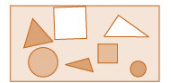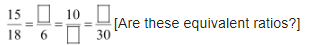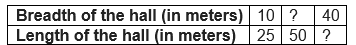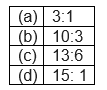# Ratio and proportion Exercise 12.1

In this page we have NCERT Solutions for Class 6 Maths Chapter 12 :Ratio and proportion Exercise 12.1. Hope you like them and do not forget to like , social share and comment at the end of the page.

## Exercise 12.1

Question 1
There are 20 girls and 15 boys in a class.
(a) What is the ratio of number of girls to the number of boys?
(b)What is the ratio of number of girls to the total number of students in the class?
(a)
Number of girls : number of boys = $20 : 5= \frac {20}{ 15}= \frac {4}{3} = 4:3$
(b) Number of girls = 20
Number of boys = 15
Total number of students in the class = 35
Number of girls: total number of students in the class = $20: 35 = \frac {20}{35}= 4: 7$

Question 2
Out of 30 students in a class, 6 like football, 12 like cricket and remaining like tennis. Find the ratio of
(a) Number of students liking football to number of students liking tennis.
(b) Number of students liking cricket to total number of students.
Given
Total number of students in the class = 30
Count of students who like football = 6
Count of students who like cricket = 12
So,
Count of students who like tennis = Total number of students in the class – (Sum of students who like football and cricket)
$=30 - (6 + 12) = 12$
(a) Number of students who like football : number of students who like tennis = $6 : 12 =\frac {6}{12}= 1: 2$
(b) Number of students who like cricket: total number of students = $12: 30 = \frac {12}{30}= 2: 5$

Question 3
See the figure and find the ratio of(a) Number of triangles to the number of circles inside the rectangle.
(b) Number of squares to all the figures inside the rectangle.
(c) Number of circles to all the figures inside the rectangle.
We can count number of different figures in the given question
Count of triangles = 3
Count of circles = 2
Count of squares = 2
Total number of figures inside the rectangle = $3+2+2= 7$
(a) Number of triangles to the number of circles inside the rectangle = 3: 2
(b) Number of squares to all the figures inside the rectangle = 2: 7
(c) Number of circles to all the figures inside the rectangle = 2: 7

Question 4
Distances travelled by Hamid and Akhtar in an hour are 9 km and 12 km. Find the ratio of speed of Hamid to the speed of Akhtar.
Distance travelled by Hamid in 1 hour = 9 km
We know that
Speed is given as
$Speed= \frac {Distance}{ Timetaken}$ So, Speed of Hamid = $\frac {distance}{time} = 9 km/hr$
Similarly, Distance travelled by Akhtar in 1 hour = 12 km
Speed of Akhtar = distance/time = 12 km/hr
Ratio of speed of Hamid: Akhtar = $9: 12 = \frac {9}{12}= 3: 4$

Question 5
Fill in the following blanks:$\frac {15}{18} = \frac {5}{6} = \frac {10}{12} = \frac {25}{30}$
These ratios are equivalent

Question 6
Find the ratio of the following:
(a) 81 to 108
(b) 98 to 63
(c) 33 km to 121 km
(d) 30 minutes to 45 minutes
 a) $\frac {81}{108} = \frac {9}{12}= \frac {3}{4}$ b) $\frac {98}{63}= \frac {14}{9}$ c) $\frac {33}{121}= \frac {3}{11}$ d) $\frac {30}{45}= \frac {6}{9}= \frac {2}{3}$

Question 7
Find the ratio of the following:
(a) 30 minutes to 1.5 hours
(b) 40 cm to 1.5 m
(c) 55 paise to Re1
(d) 500 mL to 2 litres
We can only find ratio here by converting them in same units
It is always easy to convert into smaller of unit
a) 30 minutes to 1.5 hours
1.5 hours = 90 min
So, ratio = $\frac {30}{90}= \frac {1}{3}$
b) 40 cm to 1.5 m
1.5 m = 150cm
So, ratio= $\frac {40}{150} = \frac {4}{15}$
c) 55 paise to Re 1
Re 1 = 100 paise
So ratio = $\frac {55}{100} = \frac {11}{20}$
d) 500 mL to 2 litres
2 litres= 2000ml
So ratio = $\frac {500}{2000} =\frac { 1}{4}$

Question 8
In a year, Seema earns Rs 1, 50, 000 and saves Rs 50, 000. Find the ratio of
(a) Money that Seema earns to the money she saves.
(b) Money that she saves to the money she spends.
Money earned by Seema = Rs 1,50,000
Money saved by Seema= Rs. 50,000
Money spent = Amount earned by Seema - Amount saved by Seema
$= 1,50,000 - 50,000 = 1,00,000$
a) Money Seema earns: money she saves =1,50,000: 50,000 = $\frac {150}{50} = 3 :1$
b) Money saved: money spent = 50,000: 1,00,000 = 1:2
Question 9
There are 102 teachers in a school of 3300 students. Find the ratio of the number of teachers to the number of students.
Number of teachers in the school = 102
Number of students in the school = 3300
Number of teachers: number of students =$102: 3300 = \frac {51}{1650}= \frac {17}{550} = 17:550$

Question 10
In a college, out of 4320 students, 2300 are girls. Find the ratio of
(a) Number of girls to the total number of students.
(b) Number of boys to the number of girls.
(c) Number of boys to the total number of students.
Total number of students in the school = 4320
Number of girls = 2300
Number of boys = Total number of students in the school - Number of girls
$=4320 - 2300 = 2020$
a) number of girls: total number of students
$= 2300: 4320 = \frac {2300}{4320} = \frac {230}{432} = \frac {115}{216}$
b) number of boys: number of girls
$= 2020: 2300 = \frac {202}{230} = \frac {101}{115} = 101: 115$
c) number of boys: total number of students
$= 2020: 4320 = \frac {202}{432} = \frac {101}{216} = 101: 216$

Question 11
Out of 1800 students in a school, 750 opted basketballs, 800 opted cricket and remaining opted table tennis. If a student can opt only one game, find the ratio of
(a) Number of students who opted basketball to the number of students who opted table tennis.
(b) Number of students who opted cricket to the number of students opting basketball.
(c) Number of students who opted basketball to the total number of students.
Total number of students in a school = 1800
Students who opted for basketball = 750
Students who opted for cricket = 800
Students who opted for table tennis = Total number of students in a school - (Sum of students who opted Basketball and cricket)
$=1800 - (750 + 800) = 1800 - 1550 = 250$
a) Students who opted basketball: Students who opted for table tennis
$= 750: 250 = \frac {75}{25}= \frac {3}{1} = 3:1$
b) students who opted cricket: basketball
$= 800: 750 = \frac {80}{75}= 16:15$
c) students who opted basketball: total number of students
$= 750: 1800 = \frac {75}{180} = 5:12$

Question 12
Cost of a dozen pens is Rs 180 and cost of 8 ball pens is Rs 56. Find the ratio of the cost of a pen to the cost of a ball pen.
1dozen = 12 pieces
Cost of 12 pens (a dozen) is = Rs. 180
So Cost of 1 pen = 180 ÷ 12 = Rs 15
Cost of 8 ball pens = Rs. 56
Cost of 1 ball pen = 56 ÷ 8 = Rs. 7
Cost of a pen: Cost of a ball pen = 15: 7

Question 13
Consider the statement: Ratio of breadth and length of a hall is 2: 5. Complete the following table that shows some possible breadths and lengths of the hall.Breadth of a hall: length of the hall = 2: 5
To fill the missing numbers, we find the equivalent ratios.
Since 5 × 10 = 50, we find 2 × 10 = 20.
That is,
2/5 = 20/50
So 20: 50 is the second ratio.
Similarly
40: 100 is the third ratio.

Question 14
Divide 20 pens between Sheela and Sangeeta in the ratio of 3:2.
The two parts are 3 and 2. Sum of the parts is 5.
So, Sheela gets 3 parts and Sangeetha gets 2 parts out of every 5 parts.
So we can say that
Sheela gets 3/5 of the total pens and Sangeeta gets 2/5 of the total pens.
Number of pens Sheela gets = (3/5) × 20 = 12
Number of pens Sangeeta gets = (2/5) × 20 =8
Therefore, Sheela gets 12 pens and Sangeeta gets 8 pens.

Question 15
Mother wants to divide Rs 36 between her daughters Shreya and Bhoomika in the ratio of their ages. If age of Shreya is 15 years and age of Bhoomika is 12 years, find how much Shreya and Bhoomika will get.
First of all ,we need to find the ratio of the daughters age Ratio of their ages = $15: 12 =\frac {15}{12}= 5: 4$
Now as per the question ,Mother divides Rs. 36 in the ratio of their ages 5: 4
Sum of parts =5 +4= 9
So, Shreya gets 5/9 of the total amount to be divided and Bhoomika gets 4/9 of the total amount to be divided.
Money Shreya gets =$\frac {5}{9} \times 36= 20$
Amount Bhoomika gets =$\frac {4}{9} \times 36= 16$
Therefore, Shreya gets Rs. 20 and Bhoomika gets Rs. 16

Question 16
Present age of father is 42 years and that of his son is 14 years. Find the ratio of
(a) Present age of father to the present age of son.
(b) Age of the father to the age of son, when son was 12 years old.
(c) Age of father after 10 years to the age of son after 10 years.
(d) Age of father to the age of son when father was 30 years old.
Given
Present age of father = 42 years
Present age of his son = 14 years
a) Age of father: age of son = $42: 14 = \frac {42}{14}= \frac {3}{1} = 3:1$
b) When the son was 12 = (14 – 2) years old
Then father would have been 40 = (42 – 2) years old.
Age of father: Age of son = 40: 12 = 10:3
c) Age of father after 10 years = Present age + 10 = 42 + 10 = 52 years
Age of son after 10 years = 14 + 10 = 24 years
Age of father: Age of son = 52: 24 = 13:6
d) When was the father 30 years old?
Since, 42 – 30 = 12, we know that 12 years back, the father was 30 years old.
So Age of son 12 years back was = present age – 12 =14-2= 2 year
Ratio of father: Age of son = 30: 2 = 15: 1### Practice Question

Question 1 What is $\frac {1}{2} + \frac {3}{4}$ ?
A)$\frac {5}{4}$
B)$\frac {1}{4}$
C)$1$
D)$\frac {4}{5}$
Question 2 Pinhole camera produces an ?
A)An erect and small image
B)an Inverted and small image
C)An inverted and enlarged image
D)None of the above